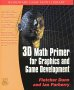# Maths - Real Normed Algebras

In the set of real numbers the square root of a minus number is a problem because it does not exist.

However, √-1can be used to generate algebras which use higher dimensional numbers, or to put it the other way round, algebras with higher dimensional numbers may have one or more solutions to the square root of a minus number.

By 'higher dimensional numbers' we mean that each element in the algebra, each number, may contain multiple real values similar to vectors. However there are a lot of possible algebras using multidimensional elements, when we are talking about real normed algebras then we define multiplication so that:

• Inverse multiplication (division) always exists, i.e. we can always find the solution to a/b
• norms are preserved by multiplication |a * b| = |a| |b|.

Where |a| = the distance from origin.

It turns out that these requirements can only be met by algebras which have 1,2,4 or 8 dimensional elements. These 4 algebras, which satisfy this condition, have the following properties:

 dimension √-1 exists Multiplication commutative Multiplication associative Real Numbers R 1 no yes yes Complex Numbers C 2 yes yes yes Quaternions Q 4 yes no yes Octonions O 8 yes no no

Complex Numbers contain a copy of the real numbers plus a copy of the real numbers multiplied by the square root of -1.

Quaternions contain a copy of the Complex Numbers plus another copy of the real numbers multiplied by another square root of -1 (going via a different dimension at 90 degrees to the first).

Octonions contain a copy of the Quaternions and double up again.

 metadata block see also: Correspondence about this page Book Shop - Further reading. Where I can, I have put links to Amazon for books that are relevant to the subject, click on the appropriate country flag to get more details of the book or to buy it from them.3D Math Primer - Aimed at complete beginners to vector and matrix algebra. Specific to this page here:

This site may have errors. Don't use for critical systems.